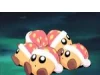## FANDOM

2,241 Pages# Thecakeofdestiny

0 Discussion posts

I am Thecakeofdestiny and people hate me for some reason.

## SignaturesIt's like looking in a mirror! - Thecakeofdestiny 23:20, February 24, 2010 (UTC)

## 1337 5934l<

4=A
8=B
<=C
l>=D
3 = E
l= = F
@ = G
l-l = H
1 = I ( may also be used for an L )
J = might be _l ( not sure )
l< = K
l_ = L ( may also be 1 )
l\/l or /\/\ = M
l/l or /\/ = N
0 = O
I think a actual P is used for P
actual Q might be used for Q
actual R may be used for R
5 = S
T might be used for a actual T
l_l = U
\/ = V
\/\/ = W
X is used for an X
Y may be used for a Y
2 Is used as a Z

Community content is available under CC-BY-SA unless otherwise noted.